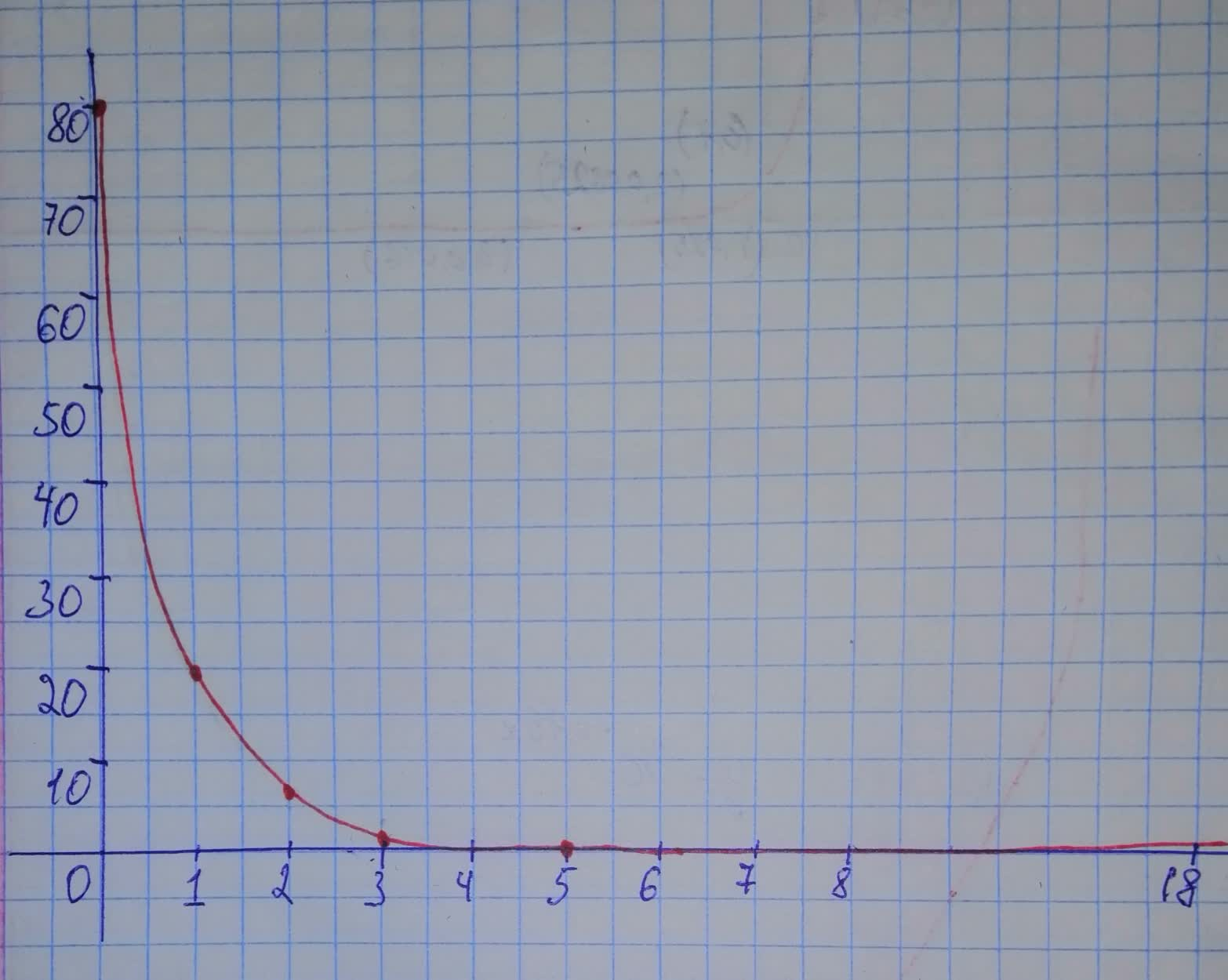# Graph each function and tell whether it represents exponential growth, exponential decay, or neither. y=80(0.25)^{x}ankarskogC 2021-01-05 Answered
Graph each function and tell whether it represents exponential growth, exponential decay, or neither.
$y=80{\left(0.25\right)}^{x}$
You can still ask an expert for help

• Questions are typically answered in as fast as 30 minutes

Solve your problem for the price of one coffee

• Math expert for every subject
• Pay only if we can solve itdelilnaT
Step 1
Graph of the function:Step 2
The function represents exponential decay because as value of x intereases, the value of y decreases. Each term has a common ratio of 0.25.
###### Not exactly what you’re looking for?Jeffrey Jordon